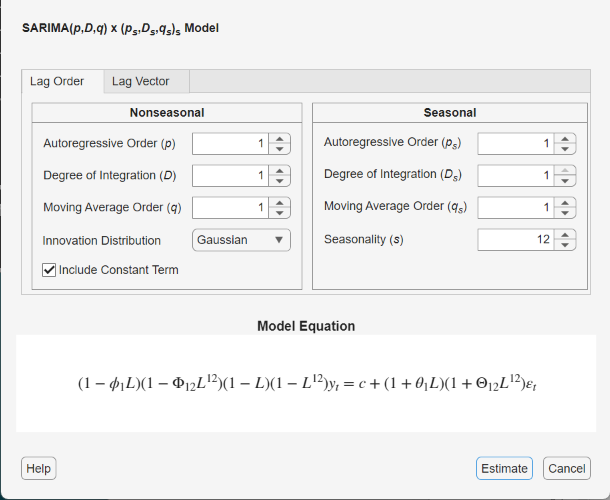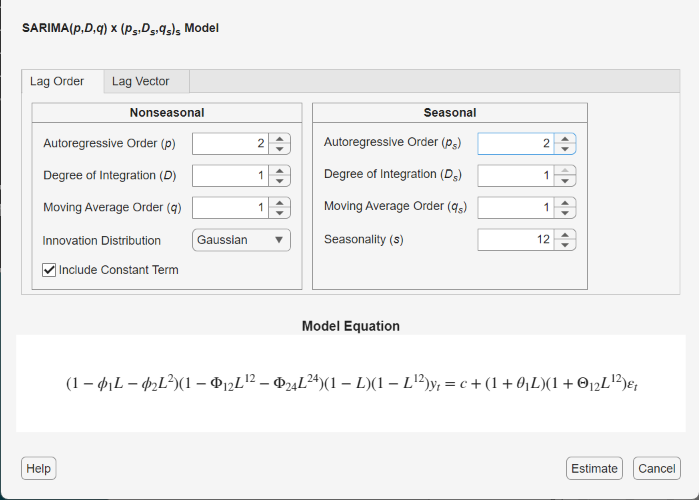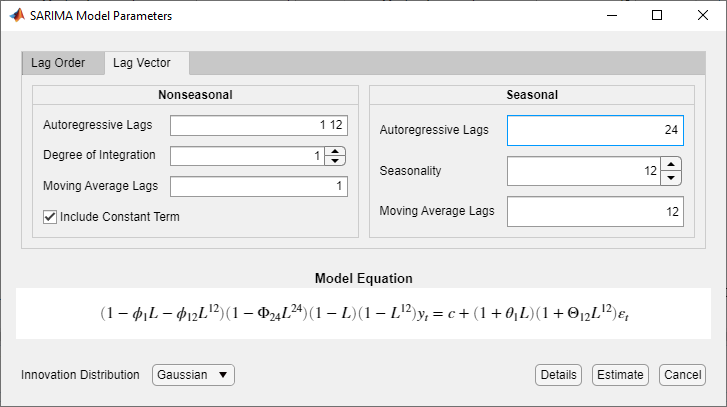## Multiplicative ARIMA Model Specifications

### Seasonal ARIMA Model with No Constant Term

This example shows how to use `arima` to specify a multiplicative seasonal ARIMA model (for monthly data) with no constant term.

Specify a multiplicative seasonal ARIMA model with no constant term,

`$\left(1-{\varphi }_{1}L\right)\left(1-{\Phi }_{12}{L}^{12}\right)\left(1-L{\right)}^{1}\left(1-{L}^{12}\right){y}_{t}=\left(1+{\theta }_{1}L\right)\left(1+{\Theta }_{12}{L}^{12}\right){\epsilon }_{t},$`

where the innovation distribution is Gaussian with constant variance. Here, $\left(1-L{\right)}^{1}$ is the first degree nonseasonal differencing operator and $\left(1-{L}^{12}\right)$ is the first degree seasonal differencing operator with periodicity 12.

```Mdl = arima('Constant',0,'ARLags',1,'SARLags',12,'D',1,... 'Seasonality',12,'MALags',1,'SMALags',12)```
```Mdl = arima with properties: Description: "ARIMA(1,1,1) Model Seasonally Integrated with Seasonal AR(12) and MA(12) (Gaussian Distribution)" Distribution: Name = "Gaussian" P: 26 D: 1 Q: 13 Constant: 0 AR: {NaN} at lag  SAR: {NaN} at lag  MA: {NaN} at lag  SMA: {NaN} at lag  Seasonality: 12 Beta: [1×0] Variance: NaN ```

The name-value pair argument `ARLags` specifies the lag corresponding to the nonseasonal AR coefficient, ${\varphi }_{1}$. `SARLags` specifies the lag corresponding to the seasonal AR coefficient, here at lag 12. The nonseasonal and seasonal MA coefficients are specified similarly. `D` specifies the degree of nonseasonal integration. `Seasonality` specifies the periodicity of the time series, for example `Seasonality` = 12 indicates monthly data. Since `Seasonality` is greater than 0, the degree of seasonal integration ${D}_{s}$ is one.

Whenever you include seasonal AR or MA polynomials (signaled by specifying `SAR` or `SMA`) in the model specification, `arima` incorporates them multiplicatively. `arima` sets the property `P` equal to p + D + ${p}_{s}$ + s (here, 1 + 1 + 12 + 12 = 26). Similarly, `arima` sets the property `Q` equal to q + ${q}_{s}$ (here, 1 + 12 = 13).

Display the value of `SAR`:

`Mdl.SAR`
```ans=1×12 cell array Columns 1 through 8 {} {} {} {} {} {} {} {} Columns 9 through 12 {} {} {} {[NaN]} ```

The `SAR` cell array returns 12 elements, as specified by `SARLags`. `arima` sets the coefficients at interim lags equal to zero to maintain consistency with MATLAB® cell array indexing. Therefore, the only nonzero coefficient corresponds to lag 12.

All of the other properties of `Mdl` are `NaN`-valued, indicating that the corresponding model parameters are estimable, or you can specify their value by using dot notation.

### Seasonal ARIMA Model with Known Parameter Values

This example shows how to specify a multiplicative seasonal ARIMA model (for quarterly data) with known parameter values. You can use such a fully specified model as an input to `simulate` or `forecast`.

Specify the multiplicative seasonal ARIMA model

`$\left(1-.5L\right)\left(1+0.7{L}^{4}\right)\left(1-L{\right)}^{1}\left(1-{L}^{4}\right){y}_{t}=\left(1+.3L\right)\left(1-.2{L}^{4}\right){\epsilon }_{t},$`

where the innovation distribution is Gaussian with constant variance 0.15. Here, $\left(1-L{\right)}^{1}$ is the nonseasonal differencing operator and $\left(1-{L}^{4}\right)$ is the first degree seasonal differencing operator with periodicity 4.

```Mdl = arima('Constant',0,'AR',0.5,'D',1,'MA',0.3,... 'Seasonality',4,'SAR',-0.7,'SARLags',4,... 'SMA',-0.2,'SMALags',4,'Variance',0.15)```
```Mdl = arima with properties: Description: "ARIMA(1,1,1) Model Seasonally Integrated with Seasonal AR(4) and MA(4) (Gaussian Distribution)" Distribution: Name = "Gaussian" P: 10 D: 1 Q: 5 Constant: 0 AR: {0.5} at lag  SAR: {-0.7} at lag  MA: {0.3} at lag  SMA: {-0.2} at lag  Seasonality: 4 Beta: [1×0] Variance: 0.15 ```

The output specifies the nonseasonal and seasonal AR coefficients with opposite signs compared to the lag polynomials. This is consistent with the difference equation form of the model. The output specifies the lags of the seasonal AR and MA coefficients using `SARLags` and `SMALags`, respectively. `D` specifies the degree of nonseasonal integration. `Seasonality` = 4 specifies quarterly data with one degree of seasonal integration.

All parameter values are specified, that is, no object property is `NaN`-valued.

### Specify Multiplicative ARIMA Model Using Econometric Modeler App

In the Econometric Modeler app, you can specify the lag structure, presence of a constant, and innovation distribution of a SARIMA(p,D,q)×(ps,Ds,qs)s model by following these steps. All specified coefficients are unknown but estimable parameters.

1. At the command line, open the Econometric Modeler app.

`econometricModeler`

Alternatively, open the app from the apps gallery (see Econometric Modeler).

2. In the Time Series pane, select the response time series to which the model will be fit.

3. On the Econometric Modeler tab, in the Models section, click the arrow to display the models gallery.

4. In the ARMA/ARIMA Models section of the gallery, click . To create SARIMAX models, see ARIMAX Model Specifications.

The SARIMA Model Parameters dialog box appears.5. Specify the lag structure. Use the Lag Order tab to specify a SARIMA(p,D,q)×(ps,Ds,qs)s model that includes:

• All consecutive lags from 1 through their respective orders, in the nonseasonal polynomials

• Lags that are all consecutive multiples of the period (s), in the seasonal polynomials

• An s-degree seasonal integration polynomial

Use the Lag Vector tab for the flexibility to specify particular lags for all polynomials. For more details, see Specifying Univariate Lag Operator Polynomials Interactively. Regardless of the tab you use, you can verify the model form by inspecting the equation in the Model Equation section.

For example, consider this SARIMA(2,1,1)×(2,1,1)12 model.

`$\left(1-{\varphi }_{1}L-{\varphi }_{2}{L}^{2}\right)\left(1-{\Phi }_{12}{L}^{12}-{\Phi }_{24}{L}^{24}\right)\left(1-L\right)\left(1-{L}^{12}\right){y}_{t}=c+\left(1+{\theta }_{1}L\right)\left(1+{\Theta }_{12}{L}^{12}\right){\epsilon }_{t},$`

where εt is a series of IID Gaussian innovations.

The model includes all consecutive AR and MA lags from 1 through their respective orders. Also, the lags of the SAR and SMA polynomials are consecutive multiples of the period from 12 through their respective specified order times 12. Therefore, use the Lag Order tab to specify the model.

1. In the Nonseasonal section:

1. Set Degree of Integration to `1`.

2. Set Autoregressive Order to `2`.

3. Set Moving Average Order to `1`.

2. In the Seasonal section:

1. Set Period to `12`.

2. Set Autoregressive Order to `2`. This input specifies the inclusion of SAR lags 12 and 24 (that is, the first and second multiples of the value of Period).

3. Set Moving Average Order to `1`. This input specifies the inclusion of SMA lag 12 (that is, the first multiple of the value of Period).

4. Select the Include Seasonal Difference check box.3. Verify that the equation in the Model Equation section matches your model.

• To exclude a constant from the model and to specify that the innovations are Gaussian, follow the previous steps, and clear the Include Constant Term check box.

• To specify t-distributed innovations, follow the previous steps, and click the button, then select `t`.

For another example, consider this SARIMA(12,1,1)×(2,1,1)12 model.

`$\left(1-{\varphi }_{1}L-{\varphi }_{12}{L}^{12}\right)\left(1-{\Phi }_{24}{L}^{24}\right)\left(1-L\right)\left(1-{L}^{12}\right){y}_{t}=c+\left(1+{\theta }_{1}L\right)\left(1+{\Theta }_{12}{L}^{12}\right){\epsilon }_{t}.$`

The model does not include consecutive AR lags, and the lags of the SAR polynomial are not consecutive multiples of the period. Therefore, use the Lag Vector tab to specify this model:

1. In the SARIMA Model Parameters dialog box, click the Lag Vector tab.

2. In the Nonseasonal section:

1. Set Degree of Integration to `1`.

2. Set Autoregressive Lags to `1 12`.

3. Set Moving Average Lags to `1`.

3. In the Seasonal section:

1. Set Seasonality to `12`. The app includes a 12-degree seasonal integration polynomial.

2. Set Autoregressive Lags to `24`. This input specifies the inclusion of SAR lag 24. The input is independent of the value in the Seasonality box.

3. Set Moving Average Lags to `12`. This input specifies the inclusion of SMA lag 12. The input is independent of the value in the Seasonality box.4. Verify that the equation in the Model Equation section matches your model.

After you specify a model, click to estimate all unknown parameters in the model.